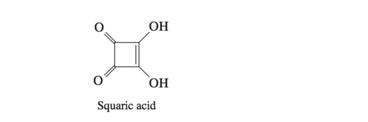# Problem: With a pKa of 1.2, squaric acid is unusually acidic for a compound containing only C, H, and O. Write a Lewis dot structure for the conjugate base of squaric acid and, using curved arrows, show how the negative charge is shared by two oxygens.

###### FREE Expert Solution
83% (217 ratings)
###### Problem Details

With a pKa of 1.2, squaric acid is unusually acidic for a compound containing only C, H, and O. Write a Lewis dot structure for the conjugate base of squaric acid and, using curved arrows, show how the negative charge is shared by two oxygens.What scientific concept do you need to know in order to solve this problem?

Our tutors have indicated that to solve this problem you will need to apply the Acid and Base Conjugates concept. If you need more Acid and Base Conjugates practice, you can also practice Acid and Base Conjugates practice problems.

What is the difficulty of this problem?

Our tutors rated the difficulty ofWith a pKa of 1.2, squaric acid is unusually acidic for a co...as low difficulty.

How long does this problem take to solve?

Our expert Organic tutor, Jonathan took 2 minutes and 24 seconds to solve this problem. You can follow their steps in the video explanation above.

What professor is this problem relevant for?

Based on our data, we think this problem is relevant for Professor Pearson's class at CASE.

What textbook is this problem found in?

Our data indicates that this problem or a close variation was asked in Organic Chemistry - Carey 8th Edition. You can also practice Organic Chemistry - Carey 8th Edition practice problems.